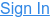Blog

# An introduction to Machine Learning

Interested in Machine Learning? This guide will introduce you to the topic, defining the basic concepts you need to know before digging deeper.

### Artificial Intelligence and Machine Learning

Artificial Intelligence, or AI, is often defined as the making of intelligent machines. In reality, it is an umbrella term describing software systems able to perform tasks that traditionally require human intervention.

AI systems are able to interact with their environment, analyzing it using a set of pre-determined rules, and making decisions based on the results. By powering machines with this autonomy, we’re building applications that were not imaginable before, like Siri, the Netflix browser, a Tesla car, or even Google.

Machine Learning is a subcategory of AI. Certain AI applications can intelligently interact with their environment, reading new data, and extracting conclusions. Still, they are not able to use what they "see" to modify themselves without the active intervention of a programmer. On the contrary, Machine Learning systems are allowed to make changes based the on information they extract from data analysis, without direct human interaction.

This is what makes machine learning special, different than other fields of computer science. ML algorithms are not only "instructions" to the computer but dynamic models that analyze how well things are working, using the information they get to improve. Machine learning is about understanding the data and the information behind it; this mathematical understanding of the data allows the machine to create models that can be used not only for describing the existing data, but for making predictions.

Machine Learning has revolutionized many aspects of computer science. Due to their complexity and inherent variability, it wouldn't be possible to write by hand the algorithms that form the core of many modern applications. AI allows computers to do what humans cannot.

### Supervised learning, unsupervised learning and reinforcement learning

Most Machine Learning problems can be divided into three main categories: supervised learning, unsupervised learning, and reinforcement learning.

##### Supervised learning

Supervised learning is the most common type of Machine Learning. In a supervised learning problem, we interact with the computer as if it was a (very smart) student; with us acting as the teacher, we give the machine some training examples that illustrate what we want it to do. Training examples are made of known pairs of related input-output data. The task of the computer is to define the mathematical function that describes the relationship between the two, using an algorithm to predict the output related to the training inputs. Then, it compares the prediction with the right answer (the known output), making adjustments until accuracy improves.

In simple words: supervised learning looks for patterns in the training data, inferring a function that describes the relationship between inputs and outputs.  Once ready, this function can be applied to new input data to make predictions. This allows not only to identify what went wrong in real-life applications, for example, but also to avoid future errors.Supervised learning problems can be grouped into regression problems (when the result we're looking for is a number) and classification problems (when we want to know if an object belongs or not to a specific class).

##### Unsupervised learning

In supervised learning, we give the computer guidance on what to look for. We have information about the relationship between the inputs and outputs, so we are able to label the data with the relevant features for the model—defining the distinguishing variables in our problem.

In some scenarios, we are not ready to do that. We might know something interesting is going on with our data, but we cannot grasp inter-relationships accurately enough to make the problem fit into a supervised learning model. We might not even be able to describe the input data correctly, since we don't know which features to look for. We need to make sense of our data first.

The role of unsupervised learning is to do precisely that: to look at unlabeled data, finding patterns and relationships that we are not able to see by ourselves. The desired result might be to group the input data into different classes (as in clustering problems) or to help clean up the data you have, discerning which features are essential for the algorithm (as in dimensionality reduction problems).Unsupervised learning models usually lead to less accurate results than the supervised learning ones, but they open the door to exciting things. Think about what this can do to the analysis of consumer behavior, to sociological studies, medical research... The computer can help us find correlations within very complex environments, information that we were totally missing before.

##### Reinforcement learning

There is a third type of Machine Learning problem. As a difference with supervised learning, it doesn't work with training examples, and unlike unsupervised learning, its goal is not to make sense of unlabeled data. Instead, reinforcement learning describes the type of Machine Learning that teaches the machine how to solve goal-oriented problems over multiple steps.

Think about game theory, for example. If a computer is playing a videogame, it will need to evaluate every move while at the same time considering the game as a whole.  The programmer cannot predict every step that will occur during the game; it is not a feasible option to give the computer if-then instructions for every situation possible.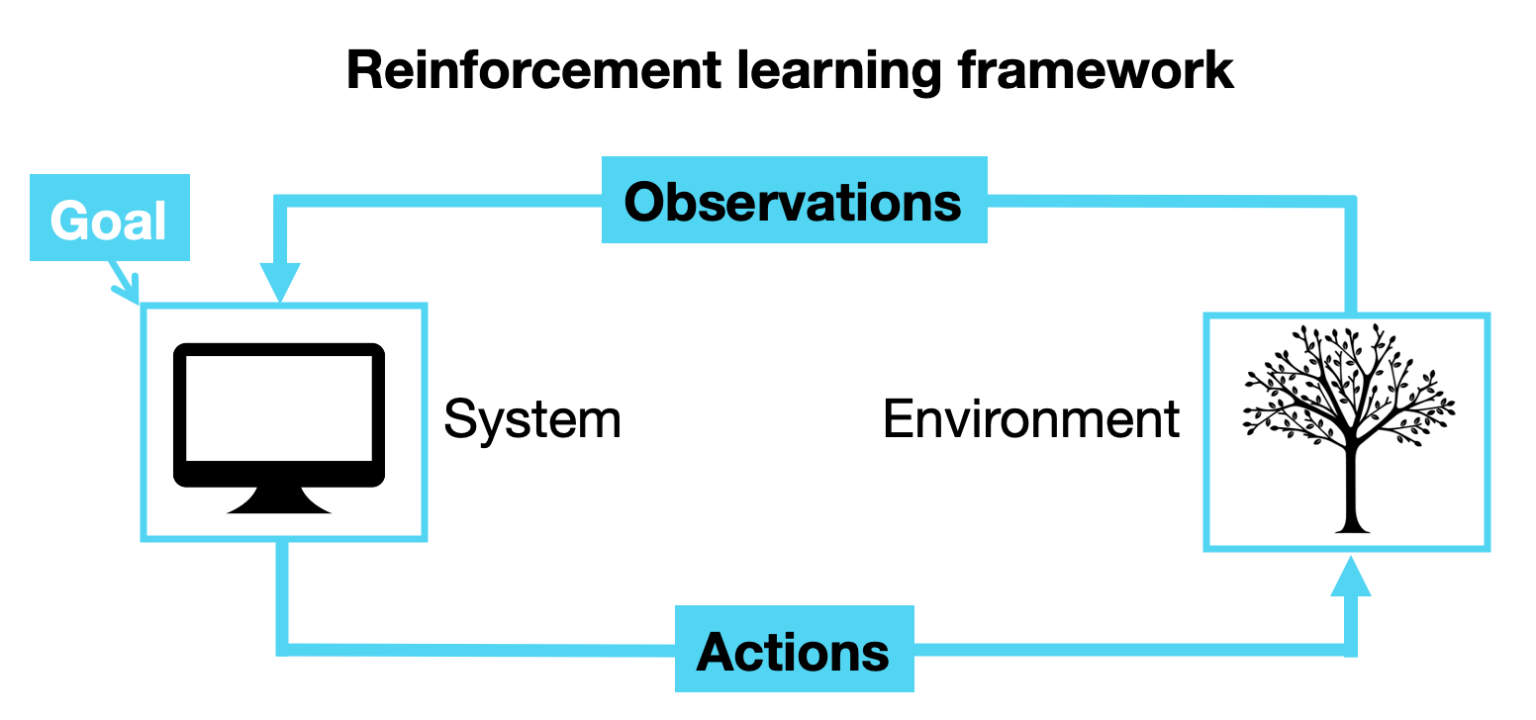Something similar happens with self-driving cars. It's not possible to describe to the computer every situation that may occur on the road with enough degree of detail, but our self-driving car will know what to do when the situation arises. By using a reinforced learning algorithm, the computer can learn how to behave by trial and error, identifying when its actions lead to a positive outcome and reinforcing that behavior within its strategy.

### Commonly used Machine Learning algorithms

Without entering into detail, let's briefly introduce some of the most common algorithms you can use to solve an ML problem. Don't forget to keep researching to learn more about them.

##### Linear regressionThe linear regression function establishes a linear relationship between the independent variable X and the dependent variable Y.  Despite its simplicity, linear regression algorithms can lead to excellent results, especially in problems with a high number of input features.

##### Logistic regression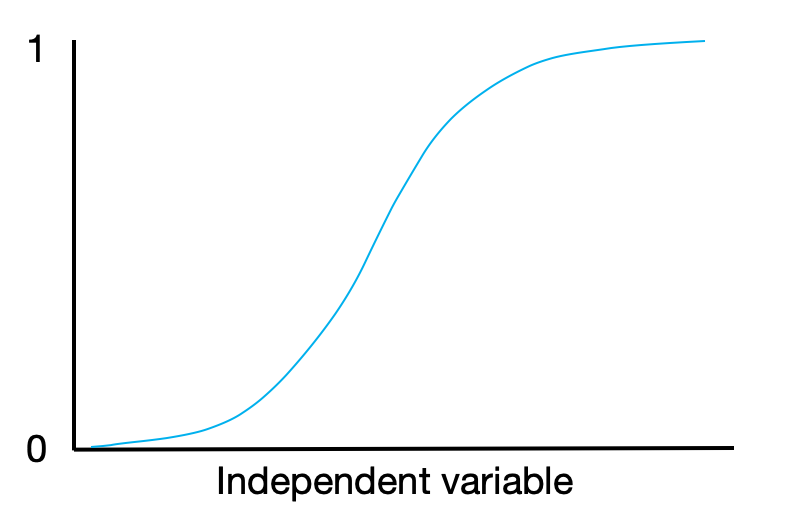Logistic regression is most suited to deal with binary classification problems, i.e., if we want to know how likely an object belongs to one or another group (yes or no, 1 or 0). It applies a classic statistic function, a sigmoid, to the independent data.

##### k-nearest neighbors (k-NN)

The k-NN algorithm can be used both for regression and classification. In both cases, k-NN looks for the k nearest neighbors to the object, with k being a parameter defined by the user. If k-NN is being used for classification, the algorithm will assign the object the most common class among the k closest neighbors. (The "closeness" is determined by a distance function.) In a regression problem, the algorithm will assign the object the average value among the k closest neighbors.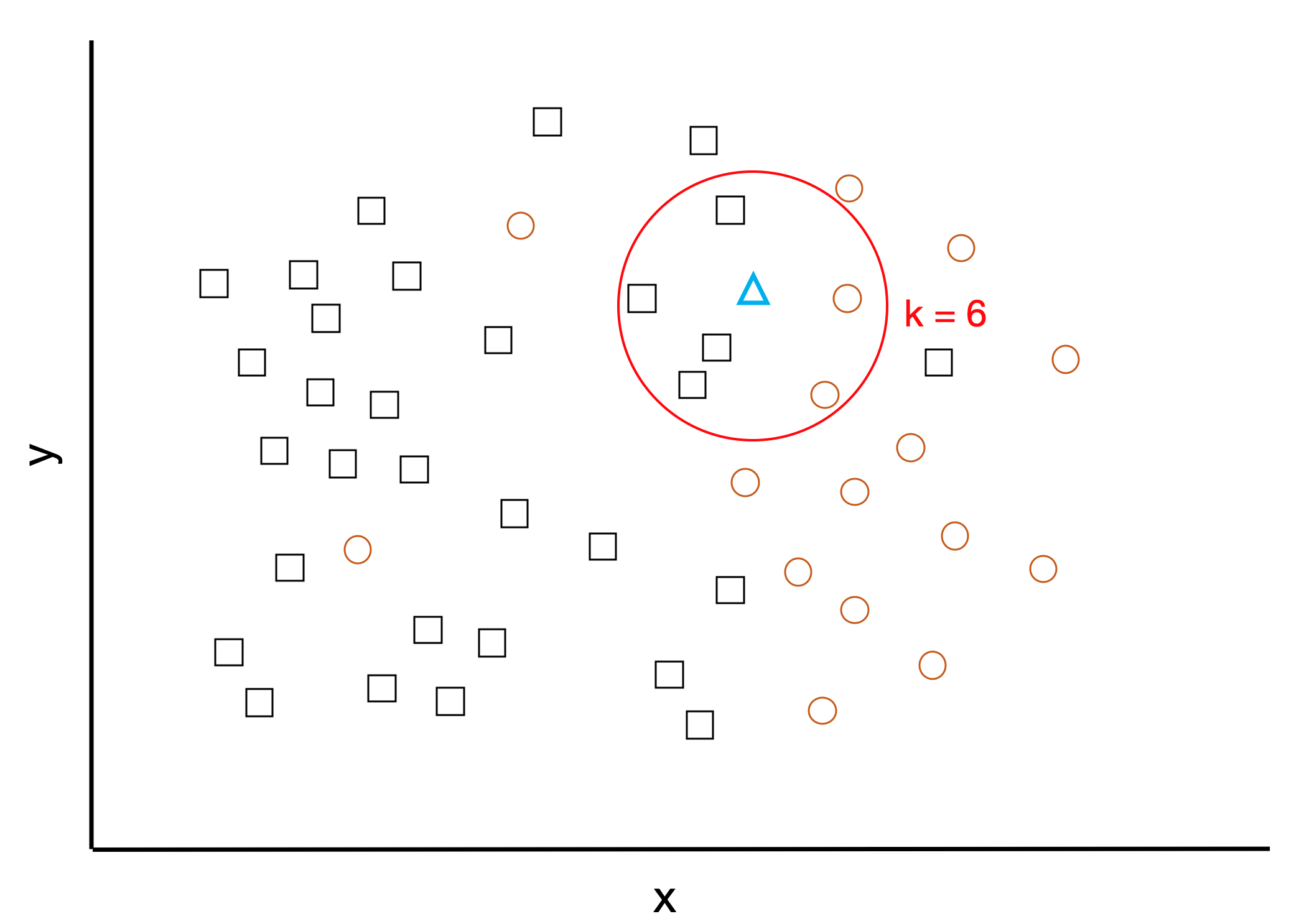To classify if the new object "triangle" belongs to the circles or squares group, k-NN looks at the 6 closest neighbors. There are 4 squares vs. 2 circles near the triangle, so it will be classified as a square.

##### k-means

k-means is mainly used for clustering problems in unsupervised learning. In k-means, k denotes the number of clusters the raw data will be finally classified into. Assuming that there's no overlapping between the clusters (every object belongs to only one group), the algorithm will divide the data into a k number of them, trying to set them up so they are as distinguishable from each other as possible.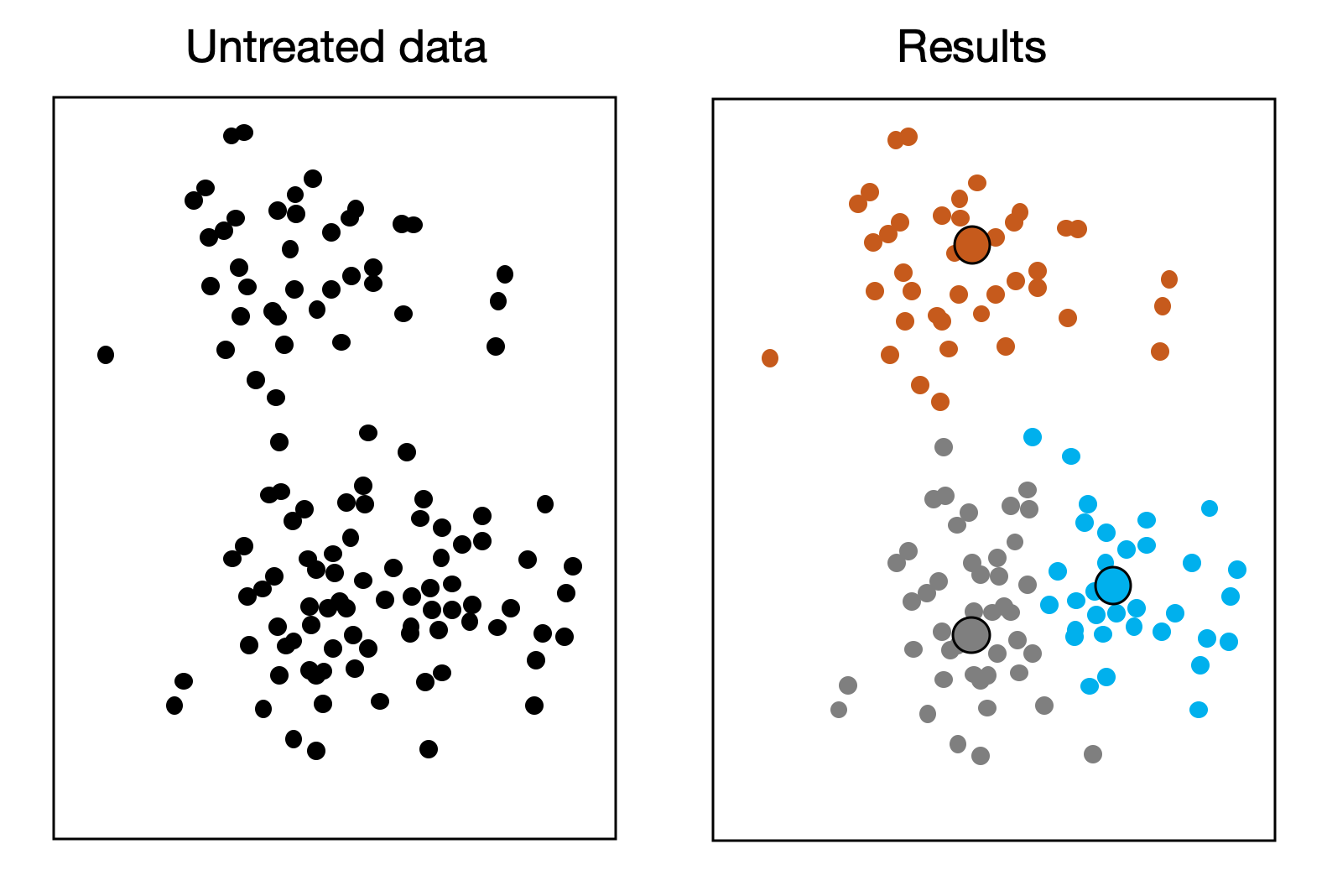The k-means algorithm classifies the raw data into a number k of clusters, assigning each object to the cluster with the nearest mean. Figure adapted from iotforall.com

##### Decision trees

Certain algorithms are suitable to be represented with a tree diagram, presenting the algorithmic process as a logical sequence that reads from top to bottom. Decision trees can be a good option for handling relatively complex classification problems, being very visual and relatively simple to follow.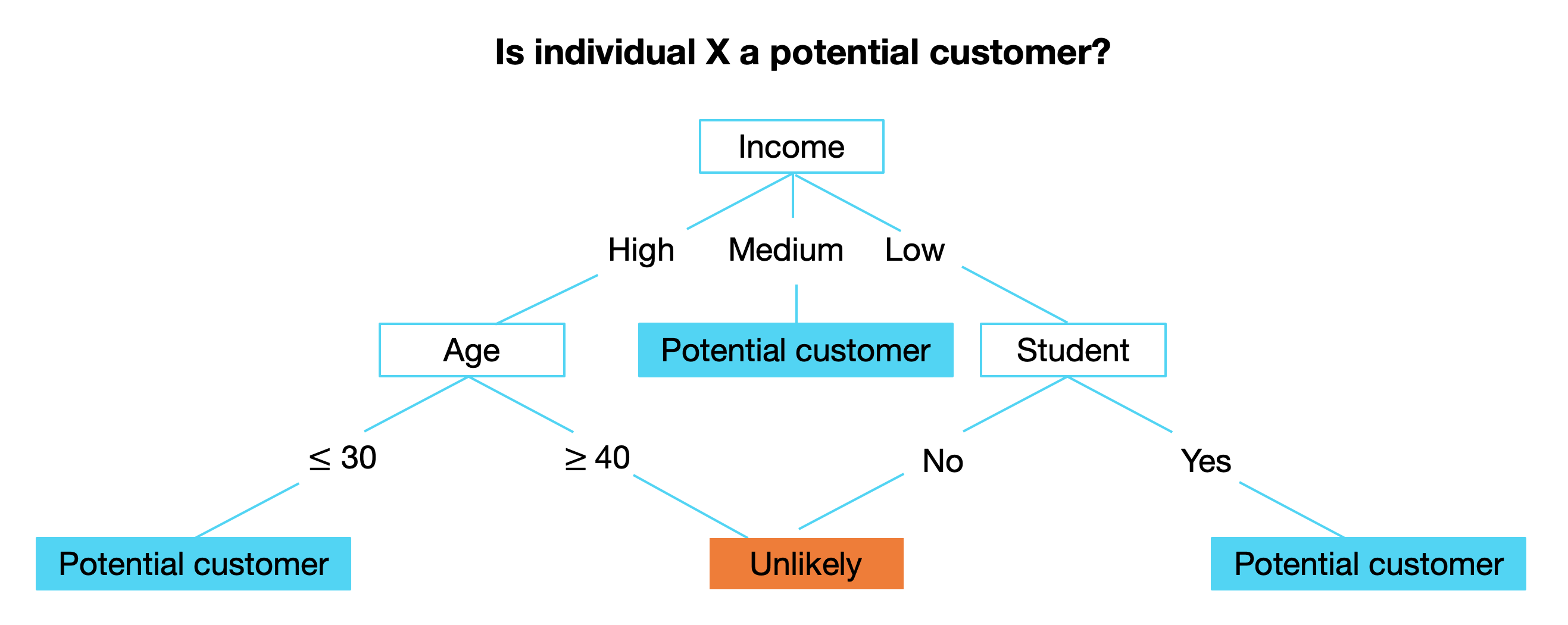A simple decision tree predicting how likely a prospect will become a customer

##### Neural networks

Neural networks are a good way of dealing with problems with a high number of inter-dependencies. These algorithms are conceptualized
as interconnected neurons that act as nodes, assigning an output to a series of inputs by applying certain mathematical functions. The neurons are combined into a network that illustrates the relationship between them, with the outputs of some being the inputs for others.

The neurons are grouped into layers, indicating how deep a specific neuron is located within the network. The "hidden layers" are the layers situated in between the external input and output layer.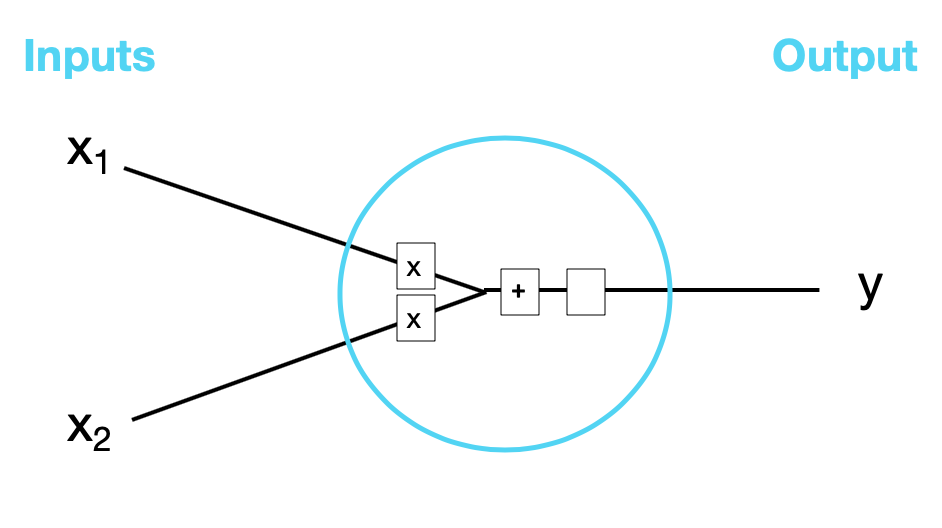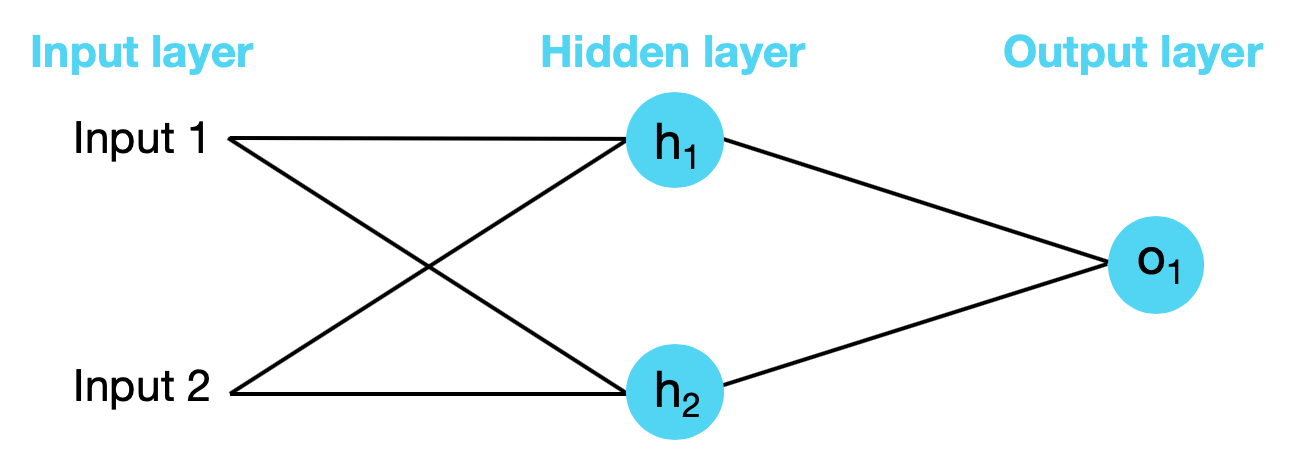A simple network with a hidden layer. Adapted from towardsdatascience.com

Neural networks are powerful tools for solving problems with complex, non-linear relationships between variables, something that more traditional models can struggle with. They can be used for regression and classification, and they get quite sophisticated. When a neural network has many layers, it's called a deep neural network. The type of Machine Learning dealing with them is referred to as deep learning.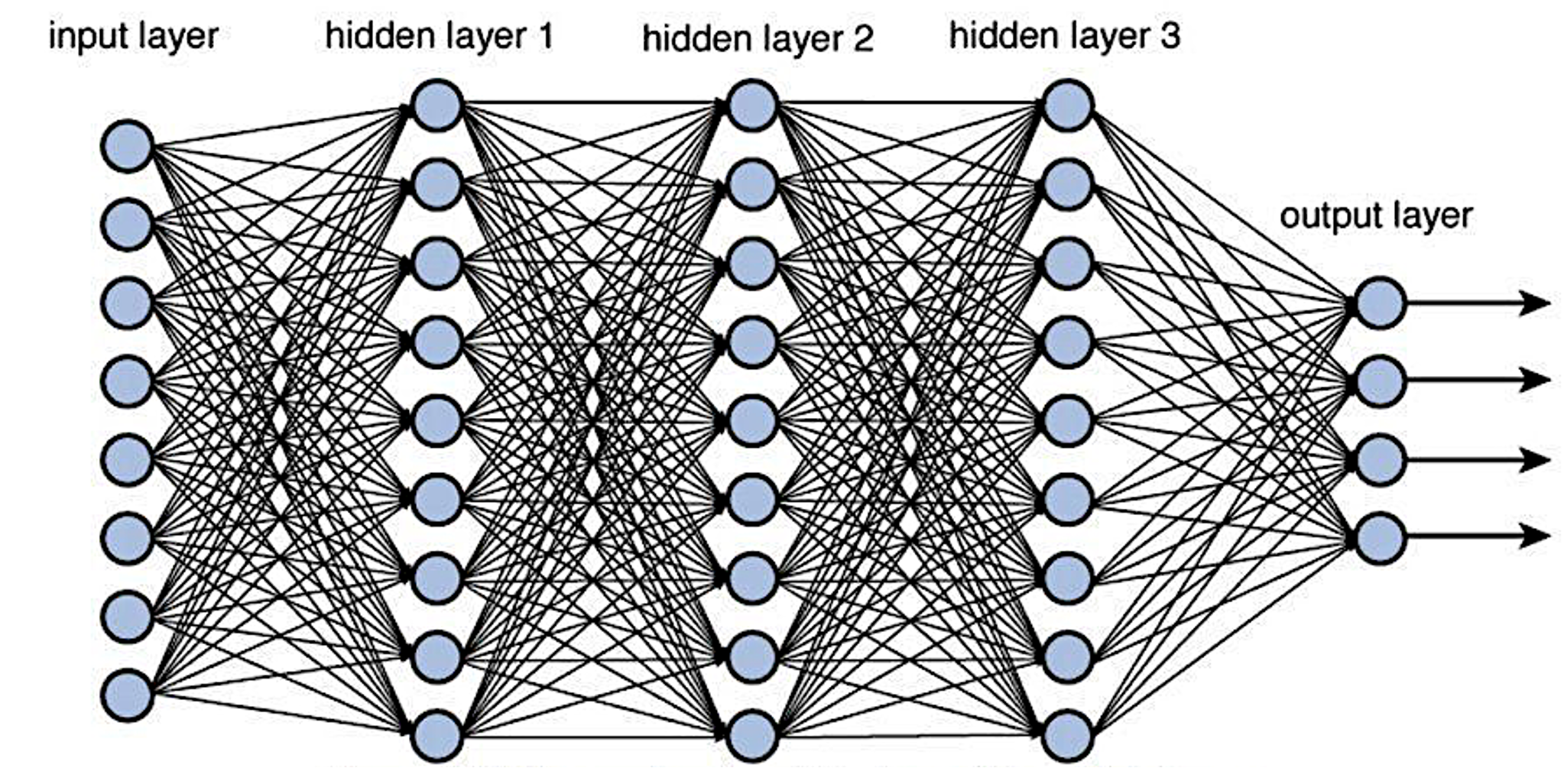Representation of a deep neural network. Source: towardsdatascience.com

##### Which algorithm should I use?

There's no one-size-fits-all for Machine Learning algorithms. There are many aspects to consider in order to determine which algorithm may work best for your problem, and to do some trial and error might be unavoidable. Some important points:

Every algorithm has been designed to fit specific problems best, so to recognize your own is the first step towards selecting the algorithm. Identify if your problem belongs to the supervised, unsupervised or reinforcement learning category, and also if you are looking at a classification or at a regression problem. You will find specific strategies for dealing with each one of these cases. Also, know your dataset as best as you can. Perform medians, averages, and percentiles to know your data range, and try to visualize information by building preliminary plots and graphs.

• If you are doing supervised learning, consider how many training examples do you have.

This is a very important factor in supervised learning. If you only have a small training dataset but you're dealing with a relatively simple case, you can probably get a good result with a high bias, low variance algorithm (for example, check out naive Bayes). However, if your problem is complex, it might be necessary to run a more "flexible" algorithm (as k-NN) over a larger training set.

• If you are doing supervised learning, pay attention to your input feature vector.

Having too much information in your input space can be detrimental to the algorithm. Make sure the features you set are relevant (and remember that there are also algorithms that can help you reduce the dimensionality of your input space). It is also important to note if your variables are inter-related. For input data with features highly independent of each other, algorithms based on linear functions tend to perform well (e.g. linear regression). The same for distance functions (e.g. k-NN). If the features interact with each other, and especially if they do it in a complex way, traditional algorithms may not lead to a good result, as we mentioned before.

Perhaps your data needs to be cleaned up, for example removing outliers (wrong data can be misleading for the algorithm) or dealing with redundancy (this is especially important for the accuracy of linear regression).  Also, consider that many traditional algorithms demand the numerical features of the input vector to be scaled to a similar range. If to include features of many different kinds and ranges is something unavoidable, it might be a good option to consider decision trees instead.

### Tutorials

Are you ready to start experimenting? In Crate.io we've built a database, CrateDB, very suitable for Machine Learning problems. Check out the following tutorials:

##### How to implement a linear regression model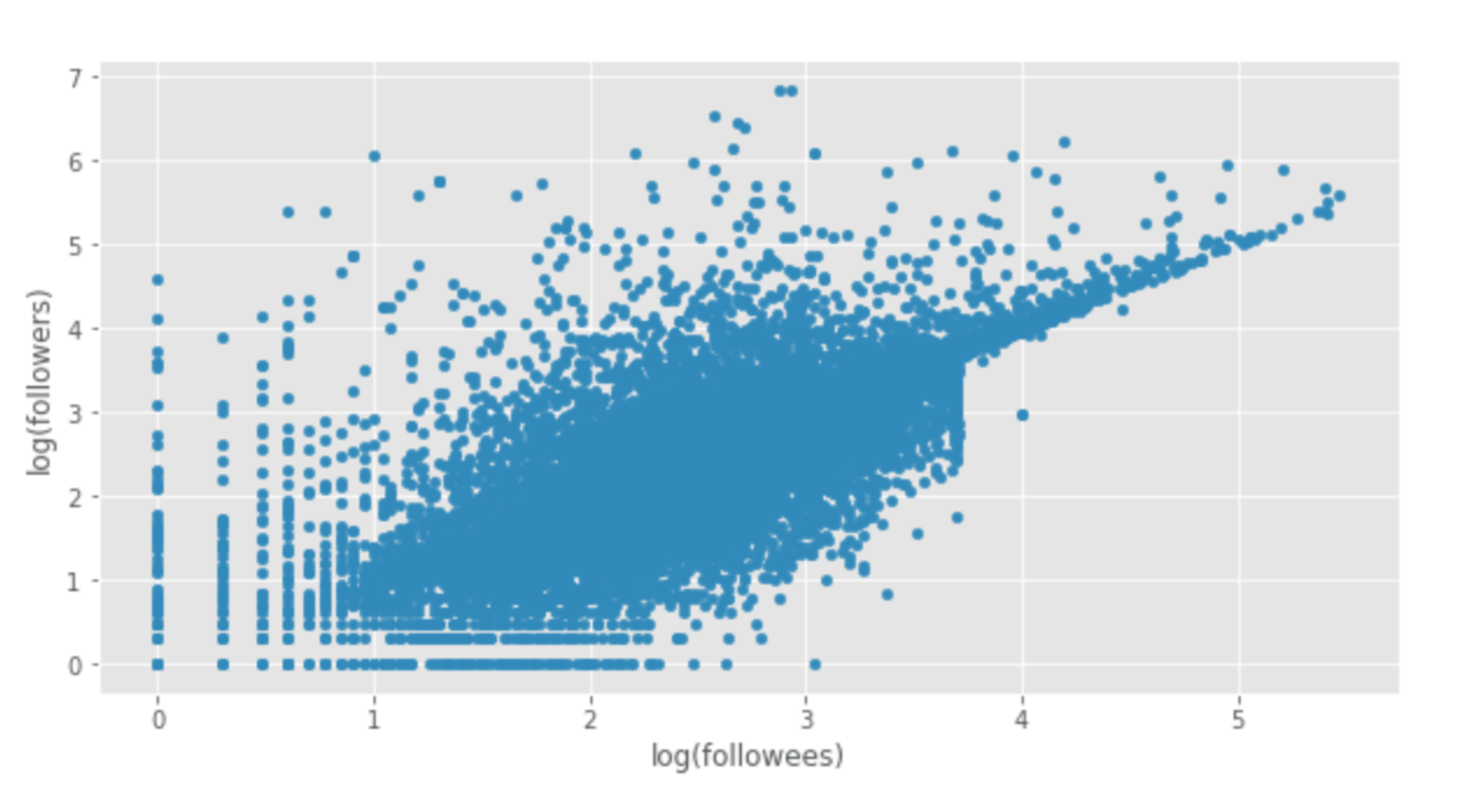This tutorial will walk you through all the steps necessary in order to apply a linear regression model.

The problem? We'll try to predict the number of followers a user has on Twitter, based on the number of people he or she follows.

As a preliminary step, go here. You'll find further context about the problem and the tools we'll be using.

##### A real-world example of applied Machine Learning: detecting turbine failures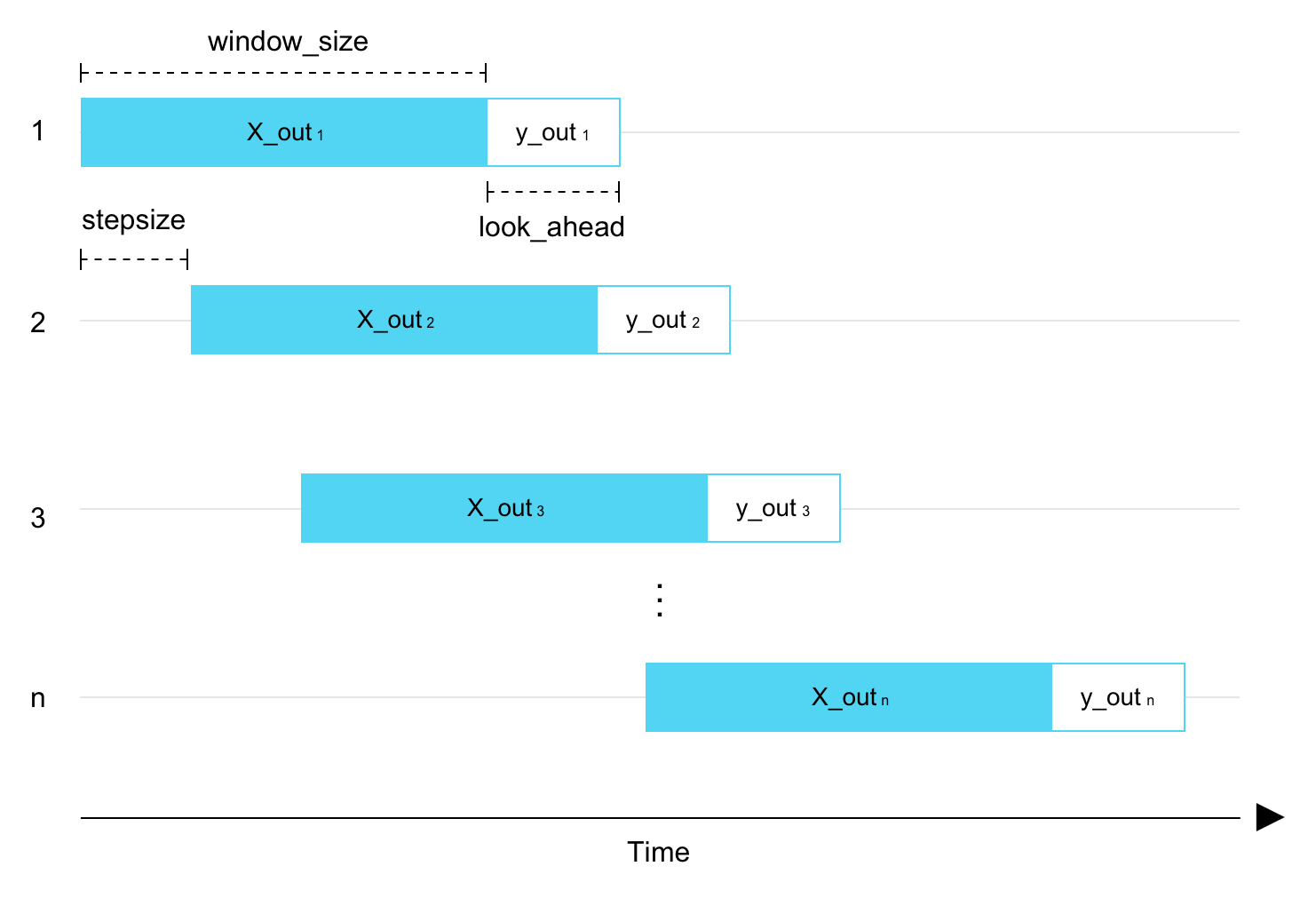In this post we show you how Machine Learning models are being applied to sensor data in a real application to predict (and prevent) critical failures.

For more context about the application (a power plant), check out the first part of the series.

##### How to implement a deep learning model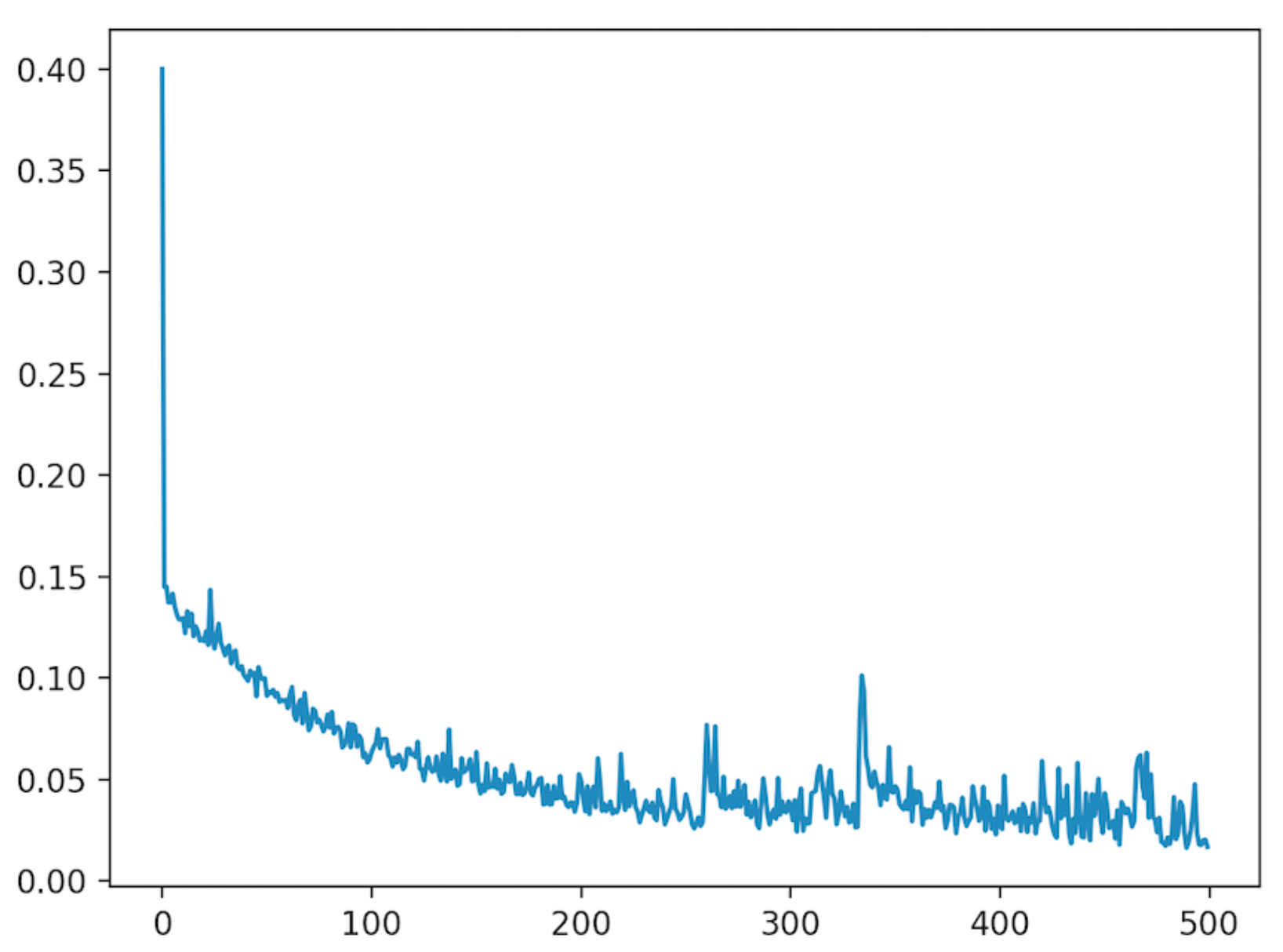This tutorial teaches you how to run a deep learning model by integrating different tools, including TensorFlow, Sklearn and CrateDB.

##### Creating a Machine Learning pipeline with CrateDB and RThis tutorial shows you how to create a Machine Learning pipeline with CrateDB and R, a language commonly used in statistical computing and data science. The tutorial walks you through the classic iris classification problem.